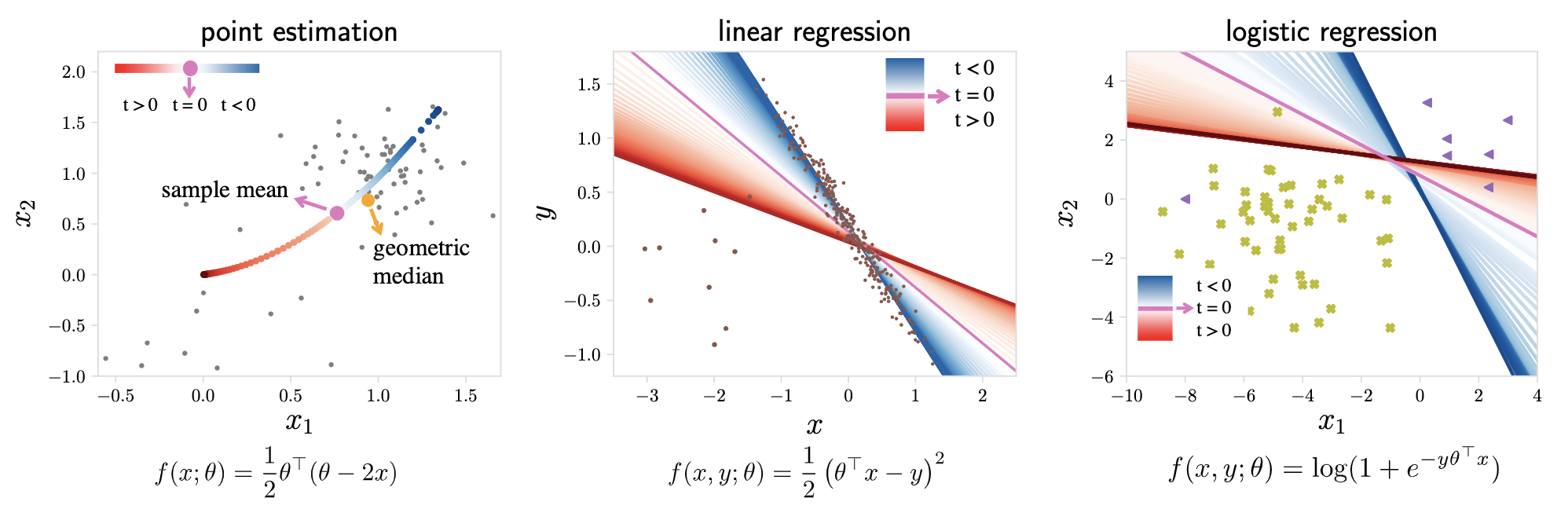/ TERM Public

Tilted Empirical Risk Minimization (ICLR '21)

# litian96/TERM

Switch branches/tags
Nothing to show

A tag already exists with the provided branch name. Many Git commands accept both tag and branch names, so creating this branch may cause unexpected behavior. Are you sure you want to create this branch?

## Files

Failed to load latest commit information.
Type
Name
Commit time

# Tilted Empirical Risk Minimization

This repository contains the implementation for the following papers

Tilted Empirical Risk Minimization, ICLR 2021

Empirical risk minimization (ERM) is typically designed to perform well on the average loss, which can result in estimators that are sensitive to outliers, generalize poorly, or treat subgroups unfairly. While many methods aim to address these problems individually, in this work, we explore them through a unified framework---tilted empirical risk minimization (TERM).

This repository contains the data, code, and experiments to reproduce our empirical results. We demonstrate that TERM can be used for a multitude of applications, such as enforcing fairness between subgroups, mitigating the effect of outliers, and handling class imbalance. TERM is not only competitive with existing solutions tailored to these individual problems, but can also enable entirely new applications, such as simultaneously addressing outliers and promoting fairness.

## Getting started

### Dependencies

As we apply TERM to a diverse set of real-world applications, the dependencies for different applications can be different.

• if we mention that the code is based on other public codebases, then one needs to follow the same setup of those codebases.
• python3
• sklearn
• numpy
• matplotlib
• colorsys
• seaborn
• scipy
• cvxpy (optional)

## Properties of TERM

### Motivating examplesThese figures illustrate TERM as a function of t: (a) finding a point estimate from a set of 2D samples, (b) linear regression with outliers, and (c) logistic regression with imbalanced classes. While positive values of t magnify outliers, negative values suppress them. Setting t=0 recovers the original ERM objective.

(How to generate these figures: cd TERM/toy_example & jupyter notebook , and directly run the three notebooks.)

### A toy problem to visualize the solutions to TERM

TERM objectives for a squared loss problem with N=3. As t moves from -to +, t-tilted losses recover min-loss (t-->+), avg-loss (t=0), and max-loss (t-->+), and approximate median-loss (for some t). TERM is smooth for all finite t and convex for positive t.

(How to generate this figure: cd TERM/properties & jupyter notebook , and directly run the notebook.)

## How to run the code for different applications

1. Robust regression

cd TERM/robust_regression
python regression.py --obj $OBJ --corrupt 1 --noise$NOISE


where $OBJ is the objective and $NOISE is the noise level (see code for options).

2. Robust classification

cd TERM/robust_classification

• built upon the public mentornet codebase
• dependencies: same as the dependencies of the mentornet codebase
• usage: see README under TERM/robust_classification

3. Mitigating noisy annotators

cd TERM/noisy_annotator/pytorch_resnet_cifar10
python trainer.py --t -2  # TERM


4. Fair PCA

cd TERM/fair_pca
jupyter notebook


and directly run the notebook fair_pca_credit.ipynb.

• built upon the public fair pca codebase
• we directly extract the pre-processed Credit data dumped from the original matlab code, which are called data.csv, A.csv, and B.csv saved under TERM/fair_pca/multi-criteria-dimensionality-reduction-master/data/credit/.
• dependencies: same as the fair pca code

5. Handling class imbalance

cd TERM/class_imbalance
python3 -m mnist.mnist_train_tilting --exp tilting  # TERM, common class=99.5%


6. Variance reduction for generalization

cd TERM/DRO
python variance_reduction.py --obj $OBJ$OTHER_PARAS


where $OBJ is the objective, and $OTHER_PARAS\$ are the hyperparameters associated with the objective (see code for options). We report how we select the hyperparameters along with all hyperparameter values in Appendix E of the paper. For instance, for TERM with t=50, run the following:

python variance_reduction.py --obj tilting --t 50


7. Fair federated learning

cd TERM/fair_flearn
bash run.sh tilting 0 0 term_t0.1_seed0 > term_t0.1_seed0 2>&1 &


8. Hierarchical multi-objective tilting

cd TERM/hierarchical
python mixed_level1.py --imbalance 1 --corrupt 1 --obj tilting --t_in -2 --t_out 10  # TERM_sc
python mixed_level2.py --imbalance 1 --corrupt 1 --obj tilting --t_in 50 --t_out -2 # TERM_ca

• mixed_level1.py: TERM_{sc}: (sample level, class level)
• mixed_level2.py: TERM_{ca}: (class level, annotator level)

## References

Please see the paper (1, 2) for more details of TERM as well as a complete list of related work.

Tilted Empirical Risk Minimization (ICLR '21)

## Releases

No releases published

## Packages 0

No packages published